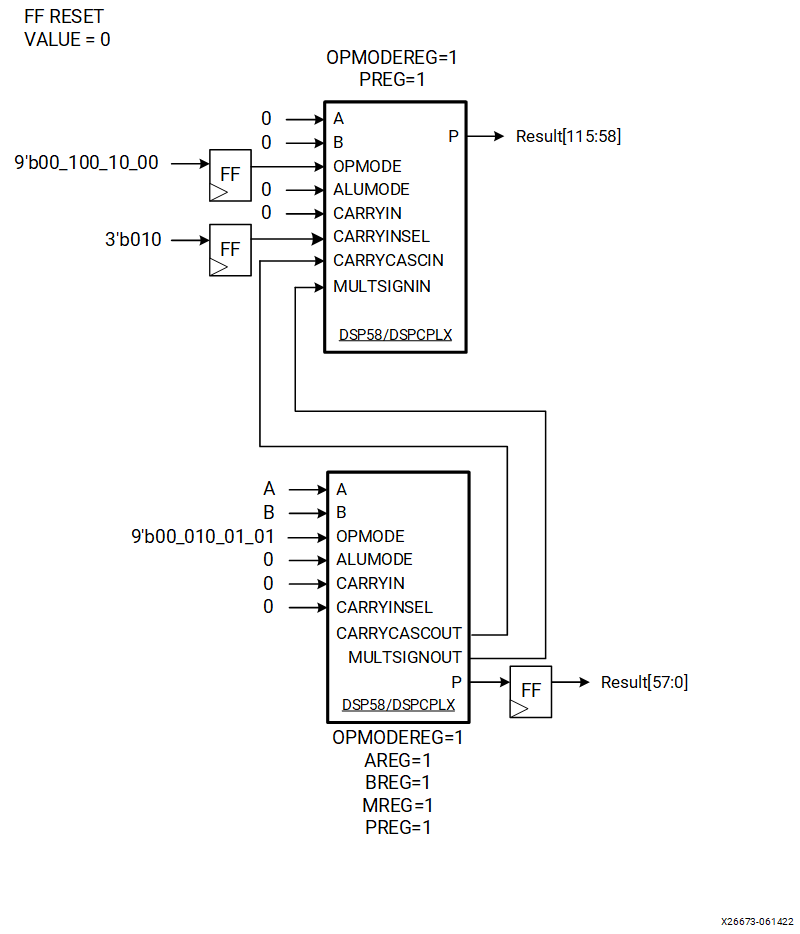# MACC Extension

## Versal ACAP DSP Engine Architecture Manual (AM004)

Document ID
AM004
Release Date
2022-09-11
Revision
1.2.1 English

A 27 x 24 bit multiplication produces a result that is representable at most on 51 bits. During MACC operations it is possible that the DSP will reach the overflow/underflow condition, with the output exceeding the available 58 bits of the DSP output (P).

Considering that the MACC operation can be written as P = P + (a × b), the number of bits necessary to represent the output result depend on the number of accumulations and can be defined as:

C ≥ log2 ( ( 2N-1 × 2M-1 × K) + 1) + 1

where
• N = number of bits for the "a" operand (represented in 2's complement)
• M = number of bits for the "b" operand (represented in 2's complement)
• K = number of accumulations
• C = number of bits for the "P" result (represented in 2's complement)

The OVERFLOW/UNDERFLOW outputs of the DSP can be used to detect the potential overflow/underflow past P. See Overflow/Underflow/Saturation for additional details.

Another approach valid only for MACC application requires the use of two DSPs as shown in the following figure.
Figure 1. MACC ApplicationThe lower DSP performs PLOWER = PLOWER + (A × B) and its output provides the lower 58 bits of the final result. The upper DSP provides the upper 58 bits of the final MACC operations, leveraging the CARRYINSEL port set to 3’b010 (selecting the CARRYCASCIN path) and an OPMODE that must be used just for this particular case: OPMODEUPPER = 9'b00_100_10_00. This OPMODE forwards the MULTSIGNIN signal to the internal ALU to provide the correct final result. It should also be selected only after the reset is de-asserted, and for this reason, OPMODEREG = 1. (During reset assertion all of the DSP internal registers are forced to zero.)

The flip-flops in the input to the upper DSP instance are required to balance the pipeline with respect to the lower DSP instance, and they are designed to output zero during reset assertion. The design proposed in the schematic shown in the previous figure provides the minimum number of registers necessary to guarantee the correct functionality of the design itself, but if additional registers are required (for example to meet timing), the following configuration will provide the expected result as well:
AREG BREG OPMODEREG CARRYINSELREG
DSP Lower Instance 2 2 1 1
DSP Upper Instance 0 0 1+1FF in logic 1+1FF in logic